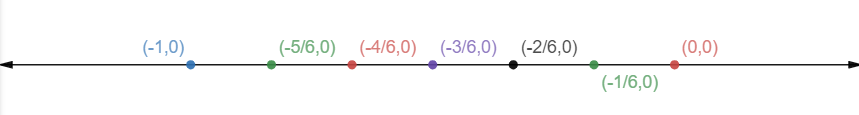Courses
Courses for Kids
Free study material
Free LIVE classes
MoreLIVE
Join Vedantu’s FREE Mastercalss

# List five rational numbers between -1 and 0.Verified
361.5k+ views
Hint: In this question we need to find rational numbers between two numbers. Here we will use a number line to solve the question.

We have to find 5 rational numbers between -1 and 0

So, we will divide -1 by 6 and then plot a number line with decreasing $\dfrac{1}{6}$.So, as we can see from the number line above, we have got 5 points between -1 and 0, which are $\dfrac{{ - 5}}{6},\dfrac{{ - 4}}{6},\dfrac{{ - 3}}{6},\dfrac{{ - 2}}{6},\dfrac{{ - 1}}{6}$

Simplifying them we get, $\dfrac{{ - 5}}{6},\dfrac{{ - 2}}{3},\dfrac{{ - 1}}{2},\dfrac{{ - 1}}{3},\dfrac{{ - 1}}{6}$

Note: Whenever we face such types of problems the main point to remember is that we need to have a good grasp over rational numbers and number lines. In these types of questions, we should always make a number line and then proceed with the question. This helps in getting us the required condition and gets us on the right track to reach the answer.
Last updated date: 27th Sep 2023
Total views: 361.5k
Views today: 6.61k